##### The Japanese Maple specialist
Direct order Contact Help / Services Newsletter# Shelf to displaySearch

Japanese MaplesBonsai soil and fertiliserAcer seeds and more Young maples and bonsaiHostasWind bells and chimesSaxifragesVarieties introduced into Europe

Packing and shipping charges

# Saxifraga fortunei beni seto midori

Saxifraga fortunei seto midori daimonjiso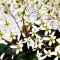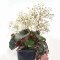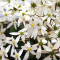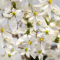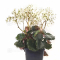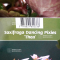ref. : 10257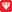11,50

Available quantity : 21Order

###### Description

Small vivacious plant to the brilliant thick leaves of Japanese origin. Flowering of mid October to end November according to the varieties and the arrival of the cold weather. Flowers doing recall the shape of the kanji dai from where does its name come[Daimonjisô]. Abundant flowering in panicles on shaft of 15 /25 centimeters of height. Inflorescence composed of 3 oval superior petals of small dimension and 2 inferior a lot stretched out. Height of 10 has 25 cm according to varieties.

Culture: Ask for an exhibition mid-shaded to shady in soil slightly acidic cool humifère and drained well. Not to let water stagnate to the foot of the plant nor the heaps of dead leaves that would encourage the rot of the collar. Can be cultivated in pot (culture in cool piece as the cyclamen hivernage out frost) in kusamono to accompany the bonsaï, but also in plates, on low walls and in full earth. This species resists has temperatures of -15 to-18 degrees on soil draining us cultivate it since more of 10 years in our nursery of Raising with success. The saxifrages loses in winter their leaves that start again in the spring.

#saxifrages 4 #leaves 3.6 #according 2.9 #varieties 2.9 #flowering 2.9 #saxifraga 2.9 #fortunei 2.8 #culture 2.7 #midori 2.6 #height 2.6

Formule
(( ROUND((CHAR_LENGTH(b.article_nom)-CHAR_LENGTH(REPLACE(b.article_nom, 'leaves', '')))/LENGTH('leaves')) + ROUND((CHAR_LENGTH(b.article_description)-CHAR_LENGTH(REPLACE(b.article_description, 'leaves', '')))/LENGTH('leaves')) ) * 3.6) + (( ROUND((CHAR_LENGTH(b.article_nom)-CHAR_LENGTH(REPLACE(b.article_nom, 'according', '')))/LENGTH('according')) + ROUND((CHAR_LENGTH(b.article_description)-CHAR_LENGTH(REPLACE(b.article_description, 'according', '')))/LENGTH('according')) ) * 2.9) + (( ROUND((CHAR_LENGTH(b.article_nom)-CHAR_LENGTH(REPLACE(b.article_nom, 'varieties', '')))/LENGTH('varieties')) + ROUND((CHAR_LENGTH(b.article_description)-CHAR_LENGTH(REPLACE(b.article_description, 'varieties', '')))/LENGTH('varieties')) ) * 2.9) + (( ROUND((CHAR_LENGTH(b.article_nom)-CHAR_LENGTH(REPLACE(b.article_nom, 'flowering', '')))/LENGTH('flowering')) + ROUND((CHAR_LENGTH(b.article_description)-CHAR_LENGTH(REPLACE(b.article_description, 'flowering', '')))/LENGTH('flowering')) ) * 2.9) + (( ROUND((CHAR_LENGTH(b.article_nom)-CHAR_LENGTH(REPLACE(b.article_nom, 'saxifraga', '')))/LENGTH('saxifraga')) + ROUND((CHAR_LENGTH(b.article_description)-CHAR_LENGTH(REPLACE(b.article_description, 'saxifraga', '')))/LENGTH('saxifraga')) ) * 2.9) + (( ROUND((CHAR_LENGTH(b.article_nom)-CHAR_LENGTH(REPLACE(b.article_nom, 'fortunei', '')))/LENGTH('fortunei')) + ROUND((CHAR_LENGTH(b.article_description)-CHAR_LENGTH(REPLACE(b.article_description, 'fortunei', '')))/LENGTH('fortunei')) ) * 2.8) + (( ROUND((CHAR_LENGTH(b.article_nom)-CHAR_LENGTH(REPLACE(b.article_nom, 'culture', '')))/LENGTH('culture')) + ROUND((CHAR_LENGTH(b.article_description)-CHAR_LENGTH(REPLACE(b.article_description, 'culture', '')))/LENGTH('culture')) ) * 2.7) + (( ROUND((CHAR_LENGTH(b.article_nom)-CHAR_LENGTH(REPLACE(b.article_nom, 'midori', '')))/LENGTH('midori')) + ROUND((CHAR_LENGTH(b.article_description)-CHAR_LENGTH(REPLACE(b.article_description, 'midori', '')))/LENGTH('midori')) ) * 2.6) + (( ROUND((CHAR_LENGTH(b.article_nom)-CHAR_LENGTH(REPLACE(b.article_nom, 'height', '')))/LENGTH('height')) + ROUND((CHAR_LENGTH(b.article_description)-CHAR_LENGTH(REPLACE(b.article_description, 'height', '')))/LENGTH('height')) ) * 2.6) + (( ROUND((CHAR_LENGTH(b.article_nom)-CHAR_LENGTH(REPLACE(b.article_nom, 'small', '')))/LENGTH('small')) + ROUND((CHAR_LENGTH(b.article_description)-CHAR_LENGTH(REPLACE(b.article_description, 'small', '')))/LENGTH('small')) ) * 2.5)

## Secure payment## Delivery

Our logistic partners :